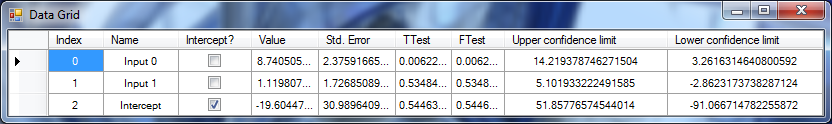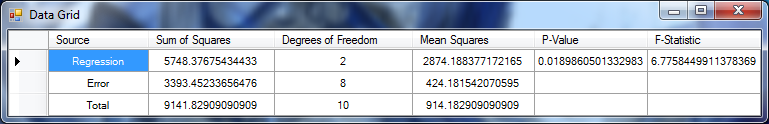﻿ MultipleLinearRegressionAnalysis Class# MultipleLinearRegressionAnalysis Class

Multiple Linear Regression AnalysisInheritance Hierarchy
SystemObject
Accord.MachineLearningTransformBaseDouble, Double
Accord.Statistics.AnalysisMultipleLinearRegressionAnalysis

Namespace:  Accord.Statistics.Analysis
Assembly:  Accord.Statistics (in Accord.Statistics.dll) Version: 3.8.0Syntax
```[SerializableAttribute]
public class MultipleLinearRegressionAnalysis : TransformBase<double[], double>,
IRegressionAnalysis, IMultivariateAnalysis, IAnalysis, IAnova, ISupervisedLearning<MultipleLinearRegression, double[], double>```

The MultipleLinearRegressionAnalysis type exposes the following members.Constructors
NameDescriptionMultipleLinearRegressionAnalysis(Boolean)
Constructs a Multiple Linear Regression Analysis.MultipleLinearRegressionAnalysis(Double, Double, Boolean) Obsolete.
Constructs a Multiple Linear Regression Analysis.MultipleLinearRegressionAnalysis(Double, Double, String, String, Boolean) Obsolete.
Constructs a Multiple Linear Regression Analysis.
TopProperties
NameDescriptionArray Obsolete.
Source data used in the analysis.ChiSquareTest
Gets a Chi-Square Test between the expected outputs and the results.Coefficients
Gets the collection of coefficients of the model.CoefficientValues
Gets the value of each coefficient.Confidences
Gets the Confidence Intervals (C.I.) for each coefficient found in the regression.FTest
Gets a F-Test between the expected outputs and results.InformationMatrix
Gets the information matrix obtained during learning.Inputs
Gets or sets the name of the input variables for the model.NumberOfInputs
Gets the number of inputs accepted by the model.
(Inherited from TransformBaseTInput, TOutput.)NumberOfOutputs
Gets the number of outputs generated by the model.
(Inherited from TransformBaseTInput, TOutput.)NumberOfSamples
Gets the number of samples used to compute the analysis.OrdinaryLeastSquares
Gets or sets the learning algorithm used to learn the MultipleLinearRegression.Output
Gets or sets the name of the output variable for the model.Outputs Obsolete.
Gets the dependent variable value for each of the source input points.Regression
Gets the Regression model created and evaluated by this analysis.Results Obsolete.
Gets the resulting values obtained by the linear regression model.RSquareAdjustedRSquared
Gets the coefficient of determination, as known as R²Source Obsolete.
Source data used in the analysis.StandardError
Gets the standard deviation of the errors.StandardErrors
Gets the Standard Error for each coefficient found during the logistic regression.Table
Gets the ANOVA table for the analysis.Token
Gets or sets a cancellation token that can be used to stop the learning algorithm while it is running.ZTest
Gets a Z-Test between the expected outputs and the results.
TopMethods
NameDescriptionCompute Obsolete.
Computes the Multiple Linear Regression Analysis.Equals
Determines whether the specified object is equal to the current object.
(Inherited from Object.)Finalize
Allows an object to try to free resources and perform other cleanup operations before it is reclaimed by garbage collection.
(Inherited from Object.)GetConfidenceInterval
Gets the confidence interval for a given input.GetHashCode
Serves as the default hash function.
(Inherited from Object.)GetPredictionInterval
Gets the prediction interval for a given input.GetType
Gets the Type of the current instance.
(Inherited from Object.)Learn
Learns a model that can map the given inputs to the given outputs.MemberwiseClone
Creates a shallow copy of the current Object.
(Inherited from Object.)ToString
Returns a string that represents the current object.
(Inherited from Object.)Transform(TInput)
Applies the transformation to a set of input vectors, producing an associated set of output vectors.
(Inherited from TransformBaseTInput, TOutput.)Transform(Double)
Applies the transformation to an input, producing an associated output.
(Overrides TransformBaseTInput, TOutputTransform(TInput).)Transform(TInput, TOutput)
Applies the transformation to an input, producing an associated output.
(Inherited from TransformBaseTInput, TOutput.)
TopExtension Methods
NameDescriptionHasMethod
Checks whether an object implements a method with the given name.
(Defined by ExtensionMethods.)IsEqual
Compares two objects for equality, performing an elementwise comparison if the elements are vectors or matrices.
(Defined by Matrix.)To(Type)Overloaded.
Converts an object into another type, irrespective of whether the conversion can be done at compile time or not. This can be used to convert generic types to numeric types during runtime.
(Defined by ExtensionMethods.)ToTOverloaded.
Converts an object into another type, irrespective of whether the conversion can be done at compile time or not. This can be used to convert generic types to numeric types during runtime.
(Defined by ExtensionMethods.)
TopRemarks

Linear regression is an approach to model the relationship between a single scalar dependent variable y and one or more explanatory variables x. This class uses a MultipleLinearRegression to extract information about a given problem, such as confidence intervals, hypothesis tests and performance measures.

This class can also be bound to standard controls such as the DataGridView by setting their DataSource property to the analysis' Coefficients property.

References:

• Wikipedia contributors. "Linear regression." Wikipedia, The Free Encyclopedia, 4 Nov. 2012. Available at: http://en.wikipedia.org/wiki/Linear_regressionExamples

The first example shows how to learn a multiple linear regression analysis from a dataset already given in matricial form (using jagged double[][] arrays).

```// And also extract other useful information, such
// as the linear coefficients' values and std errors:
double[] coef = mlra.CoefficientValues;
double[] stde = mlra.StandardErrors;

// Coefficients of performance, such as r²
double rsquared = mlra.RSquared; // 0.62879

// Hypothesis tests for the whole model
ZTest ztest = mlra.ZTest; // 0.99999
FTest ftest = mlra.FTest; // 0.01898

// and for individual coefficients
TTest ttest0 = mlra.Coefficients.TTest; // 0.00622
TTest ttest1 = mlra.Coefficients.TTest; // 0.53484

// and also extract confidence intervals
DoubleRange ci = mlra.Coefficients.Confidence; // [3.2616, 14.2193]

// We can use the analysis to predict an output for a sample
double y = mlra.Regression.Transform(new double[] { 10, 15 });

// We can also obtain confidence intervals for the prediction:
DoubleRange pci = mlra.GetConfidenceInterval(new double[] { 10, 15 });

// and also prediction intervals for the same prediction:
DoubleRange ppi = mlra.GetPredictionInterval(new double[] { 10, 15 });```
```// Now we can show a summary of analysis
// Accord.Controls.DataGridBox.Show(regression.Coefficients);``````// We can also show a summary ANOVA
DataGridBox.Show(regression.Table);``````// And also extract other useful information, such
// as the linear coefficients' values and std errors:
double[] coef = mlra.CoefficientValues;
double[] stde = mlra.StandardErrors;

// Coefficients of performance, such as r²
double rsquared = mlra.RSquared; // 0.62879

// Hypothesis tests for the whole model
ZTest ztest = mlra.ZTest; // 0.99999
FTest ftest = mlra.FTest; // 0.01898

// and for individual coefficients
TTest ttest0 = mlra.Coefficients.TTest; // 0.00622
TTest ttest1 = mlra.Coefficients.TTest; // 0.53484

// and also extract confidence intervals
DoubleRange ci = mlra.Coefficients.Confidence; // [3.2616, 14.2193]

// We can use the analysis to predict an output for a sample
double y = mlra.Regression.Transform(new double[] { 10, 15 });

// We can also obtain confidence intervals for the prediction:
DoubleRange pci = mlra.GetConfidenceInterval(new double[] { 10, 15 });

// and also prediction intervals for the same prediction:
DoubleRange ppi = mlra.GetPredictionInterval(new double[] { 10, 15 });```

The second example shows how to learn a multiple linear regression analysis using data given in the form of a System.Data.DataTable. This data is also heterogeneous, mixing both discrete (symbol) variables and continuous variables. This example is also available for LogisticRegressionAnalysis.

```// Note: this example uses a System.Data.DataTable to represent input data,
// but note that this is not required. The data could have been represented
// as jagged double matrices (double[][]) directly.

// If you have to handle heterogeneus data in your application, such as user records
// in a database, this data is best represented within the framework using a .NET's
// DataTable object. In order to try to learn a classification or regression model
// using this datatable, first we will need to convert the table into a representation
// that the machine learning model can understand. Such representation is quite often,
// a matrix of doubles (double[][]).
var data = new DataTable("Customer Revenue Example");

data.Columns.Add("Day", "CustomerId", "Time (hour)", "Weather", "Revenue");

// One way to perform this conversion is by using a Codification filter. The Codification
// filter can take care of converting variables that actually denote symbols (i.e. the
// weather in the example above) into representations that make more sense given the assumption
// of a real vector-based classifier.

// Create a codification codebook
var codebook = new Codification()
{
{ "Weather", CodificationVariable.Categorical },
{ "Time (hour)", CodificationVariable.Continuous },
{ "Revenue", CodificationVariable.Continuous },
};

// Learn from the data
codebook.Learn(data);

// Now, we will use the codebook to transform the DataTable into double[][] vectors. Due
// the way the conversion works, we can end up with more columns in your output vectors
// than the ones started with. If you would like more details about what those columns
// represent, you can pass then as 'out' parameters in the methods that follow below.
string[] inputNames;  // (note: if you do not want to run this example yourself, you
string outputName;    // can see below the new variable names that will be generated)

// Now, we can translate our training data into integer symbols using our codebook:
double[][] inputs = codebook.Apply(data, "Weather", "Time (hour)").ToJagged(out inputNames);
double[] outputs = codebook.Apply(data, "Revenue").ToVector(out outputName);
// (note: the Apply method transform a DataTable into another DataTable containing the codified
//  variables. The ToJagged and ToVector methods are then used to transform those tables into
//  double[][] matrices and double[] vectors, respectively.

// If we would like to learn a linear regression model for this data, there are two possible
// ways depending on which aspect of the linear regression we are interested the most. If we
// are interested in interpreting the linear regression, performing hypothesis tests with the
// coefficients and performing an actual _linear regression analysis_, then we can use the
// MultipleLinearRegressionAnalysis class for this. If however we are only interested in using
// the learned model directly to predict new values for the dataset, then we could be using the
// MultipleLinearRegression and OrdinaryLeastSquares classes directly instead.

// This example deals with the former case. For the later, please see the documentation page
// for the MultipleLinearRegression class.

// We can create a new multiple linear analysis for the variables
var mlra = new MultipleLinearRegressionAnalysis(intercept: true)
{
// We can also inform the names of the new variables that have been created by the
// codification filter. Those can help in the visualizing the analysis once it is
// data-bound to a visual control such a Windows.Forms.DataGridView or WPF DataGrid:

Inputs = inputNames, // will be { "Weather: Sunny", "Weather: Rain, "Weather: Cloudy", "Time (hours)" }
Output = outputName  // will be "Revenue"
};

// To overcome linear dependency errors
mlra.OrdinaryLeastSquares.IsRobust = true;

// Compute the analysis and obtain the estimated regression
MultipleLinearRegression regression = mlra.Learn(inputs, outputs);

// And then predict the label using
double predicted = mlra.Transform(inputs); // result will be ~72.3

// Because we opted for doing a MultipleLinearRegressionAnalysis instead of a simple
// linear regression, we will have further information about the regression available:
int inputCount = mlra.NumberOfInputs;   // should be 4
int outputCount = mlra.NumberOfOutputs; // should be 1
double r2 = mlra.RSquared;              // should be 0.12801838425195311
AnovaSourceCollection a = mlra.Table;   // ANOVA table (bind to a visual control for quick inspection)
double[][] h = mlra.InformationMatrix;  // should contain Fisher's information matrix for the problem
ZTest z = mlra.ZTest;                   // should be 0 (p=0.999, non-significant)```See Also